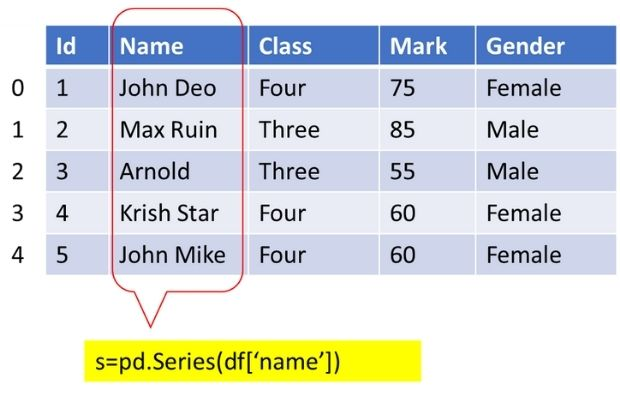# Pandas Series

Pandas

Series is one dimensional labelled data structure. ( DataFrame is two dimensional )

Series can store string, integer and other python objects. It is like one column in a table. Syntax
``````pandas.Series(data=None, index=None, dtype=None, name=None,
copy=False, fastpath=False)``````
`data` : array like , iterable , dictionary, list, tuple , scalar
`index` : have the same length as data, Not necessarily unique,must be a hashable type
`dtype` : Data type of output series
`name`: Name given to series
`copy`: Default is false, Copy input data

## Creating Series

Using List we can create one series
``````import pandas as pd
my_list=['Ravi','Raju','Alex']
s=pd.Series(data=my_list)
print(s)``````
Output
``````0    Ravi
1    Raju
2    Alex``````
We can add our index to this
``````my_list=['Ravi','Raju','Alex']
my_id=['p','q','r']
s=pd.Series(data=my_list,index=my_id)
print(s)``````
Output
``````p    Ravi
q    Raju
r    Alex``````
Using set as data source we can't create series as set is unordered.

Using tuple.
``````my_list=('Ravi','Raju','Alex')
s=pd.Series(data=my_list)
print(s)``````
Using dictionary as data source. Here dictionary keys are used as index.
``````my_dict={'a':'Ravi','b':'Raju','c':'Alex'}
s=pd.Series(data=my_dict)
print(s)``````
Output
``````a    Ravi
b    Raju
c    Alex``````
Only matching index are used.
``````my_dict={'a':'Ravi','b':'Raju','c':'Alex'}
s=pd.Series(data=my_dict,index=['a','c'])
print(s)``````
Output
``````a    Ravi
c    Alex ``````
All unmatching index
``````my_dict={'a':'Ravi','b':'Raju','c':'Alex'}
s=pd.Series(data=my_dict,index=['x','y','z'])
print(s)``````
Output
``````x    NaN
y    NaN
z    NaN``````
Using Scalar value
``````s=pd.Series(data='Alex',index=['x','y','z'])
print(s)``````
Output
``````x    Alex
y    Alex
z    Alex``````

## Using Numpy

We can create one dimensional numpy array.
``````import numpy as np
ar=np.random.rand(4) # Numpy array with random numbers
s=pd.Series(ar)
print(s)``````

## From DataFrame to SeriesFrom DataFrame we can create Series by using columns. Read more on our sample student DataFrame. This sample DataFrame has 35 rows.
``````import pandas as pd
s=pd.Series(df['name']) # using name column data
print(s)``````
We can create a Series by using all columns of a DataFrame by converting the DataFrame to a list by using tolist()
``s=pd.Series(df.values.tolist())``
Sample output is here ( few records are shown, actual output will contain 35 records )
``````0        [1, John Deo, Four, 75, female]
1         [2, Max Ruin, Three, 85, male]
2           [3, Arnold, Three, 55, male]
3      [4, Krish Star, Four, 60, female]
----
----
31    [32, Binn Rott, Seven, 90, female]
32      [33, Kenn Rein, Six, 96, female]
33       [34, Gain Toe, Seven, 69, male]
34     [35, Rows Noump, Six, 88, female]
``````

## Accessing elements of a Series

Using head() and tail() ( above code with 35 records to be used )
``````print(s.head()) # First five records
print(s.tail()) # last  five records
print(s.tail(2)) # last  two records
print(s[:5]) # first 5 records``````
Using iloc
``print(s.iloc[3:7]) ``
Output
``````3     Krish Star
4      John Mike
5      Alex John
6    My John Rob``````
Getting alternate rows
``print(s.iloc[::2]) # alternate rows ``
Using index position
``````my_dict={'a':'Ravi','b':'Raju','c':'Alex'}
s=pd.Series(data=my_dict)
print(s['b']) # Output:  Raju``````

## Updating elements of series

``````my_dict={'a':'Ravi','b':'Raju','c':'Alex'}
s=pd.Series(data=my_dict)
s['b']='King' # updating using index
print(s)``````
Output
``````a    Ravi
b    King
c    Alex``````

## Converting Data type of Series

By default this will be inferred from data. Here the id column is integer so by default the dtype is integer (`dtype: int64`) . Here we have specified the dtype to be string. Check the output by removing `dtype='string'`.
How to create sample student DataFrame?
``````df= pd.read_csv('D:\\my_data\\student.csv') # csv  file
s=pd.Series(df['id'],dtype='string')
print(s) # Name : id , dtype string``````

Subscribe to our YouTube Channel here

## Subscribe

* indicates required
Subscribe to plus2netplus2net.com